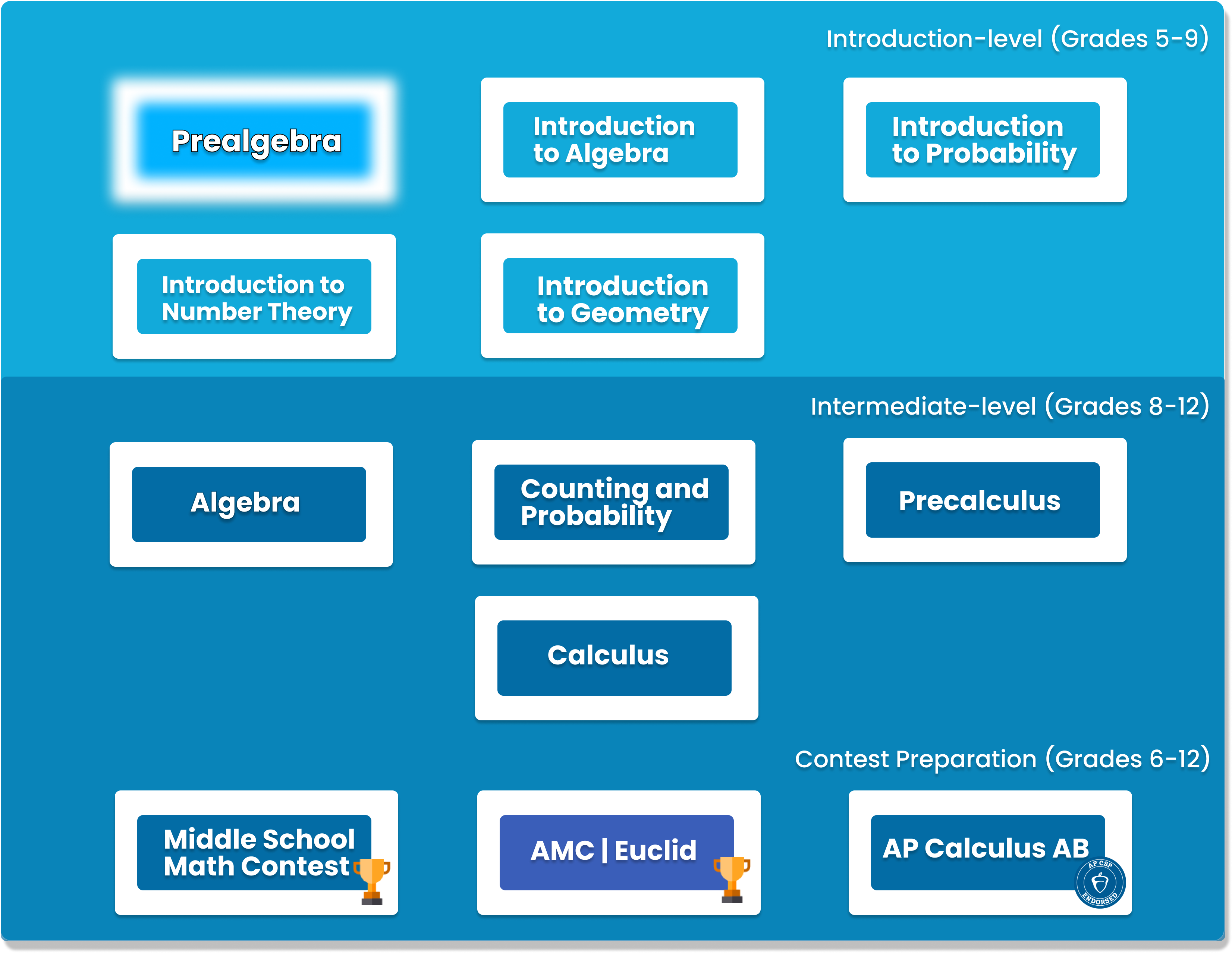# Pre-Algebra

Pre-Algebra prepares students for the rigors of Algebra studies while focusing on building problem-solving and logic techniques that will prepare them to excel in middle school. The curriculum is designed to challenge students at a deeper level than the standard middle school math concepts. Pre-Algebra offers students a well-rounded understanding of math. It covers major topics like arithmetic; divisibility, GCD, LCM; ratios rates, and percent; angles, perimeter, and area; basic statistics and counting.##### Who is this course for
This course typically takes 3-6 months to complete. This depends on the student's experience and how fast they can master the concepts and the knowledge. Our instructors move at the pace of the student, it may take extra time for some students to reinforce what they have learned.
##### Curriculum
###### Chapter 1: ArithmeticReview of addition, subtraction, multiplication, and division
###### Chapter 2: ExponentsDefinition of exponents, zero and negative exponents
###### Chapter 3: Number TheoryDivisibility, prime numbers, least common multiples, greatest common divisor
###### Chapter 4: FractionMultiplication, division, addition and subtraction of fractions
###### Chapter 5: Equations and InequalitiesLinear equations and inequalities, as well as their applications
###### Chapter 6: DecimalsArithmetic, Rounding, repeating decimals
###### Chapter 7: Ratios, Conversions, and RatesConversions of units, Word problems involving ratios and speed
###### Chapter 8: PercentDefinition of percent, percent increase and decrease, word problems
###### Chapter 9: Square RootsDefinition of square roots, arithmetic of square roots
###### Chapter 10: AnglesDefinition of angles, parallel lines and their applications
###### Chapter 11: Perimeter and AreaPerimeters and areas of shapes (rectangle, triangle, circle, etc)
###### Chapter 12: Right Triangles and Quadrilaterals###### Chapter 13: Data StatisticsMean, median of data, and visualization of data with tables, graphs, and charts
###### Chapter 14: CountingCounting with addition, multiplication principle, basic probability
###### Chapter 15: Problem-Solving StrategiesLearn to solve problems by finding patterns, drawing pictures, etc.

### You Might Also Be Interested In Our Elective Courses##### Python Level 1
AGES 10 - 15

Designed for beginners, this level is designed to teach the basic fundamentals and design principles of Python, with the help of Turtle graphics and PyGame. This includes: variables, conditional statements, loop basics, and functions.

These concepts are transferable to any other programming language. Throughout the way, students will create projects in order to apply the concepts they have learned, and to solidify their knowledge.##### Python Level 2
AGES 10 - 15

Level 2 dives deeper into the basics of Python for a more thorough understanding and introduces advanced topics. Students will build on knowledge from Level 1 and work with data structures, advanced loops, algorithms, and object-oriented programming, and create games based on what they learn.

Students will complete the course with a solid understanding of Python fundamentals.##### Java Level 1
AGES 10 - 15

This beginner-friendly course serves as an introduction to the Java programming language. Students will learn the fundamentals of Java along with core computer science concepts. Concepts covered in this course include Java syntax, types and identifiers, operators, if statements, loops, arrays and more.

Students will apply these concepts to create fun games such as Tic-Tac-Toe.